# Class 11 Maths NCERT Solutions for Chapter 5 Complex Numbers and Quadratic Equations Miscellaneous Exercise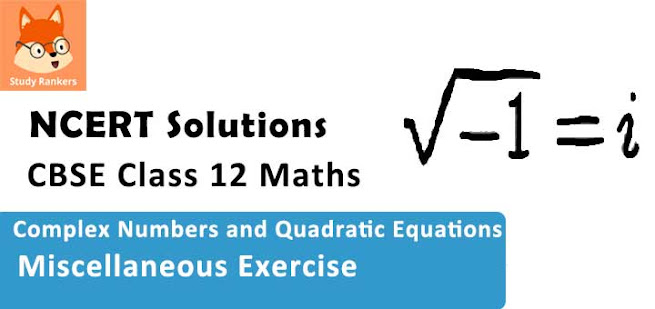### Complex Numbers and Quadratic Equations Miscellaneous Exercise Solutions

1. Evaluate : [i18 + (1/i)25 ]3 .

Solution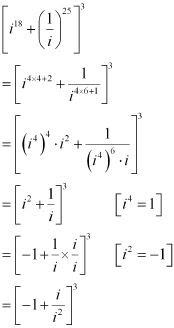= [-1 - i]3
= (-1)3 [1 +i]3
= -[13 + i3 + 3.1.i(1 + i)]
= -[1 + i3 + 3i + 3i2 ]
= -[1 - i + 3i -3]
= -[-2+2i]
= 2 -2i

2. For any two complex numbersz1 and z2, prove that
Re (z1z2)= Re zRe z2 – Im z1 Im z2
Solution
Let z1 = x1 + iy1 and z2 = x2 + iy2
∴ z1z2 = (x1 + iy1)(x2 + iy2)
= x1 (x2 + iy2) + iy1 (x2 + iy2
= x1x2 + ix1y2 + iy1x2 + i2 y1y2
= x1x2 + ix1y2 + iy1x2 + y1y2 [i2 = -1]
= (x1x2 - y1y2) + i(x1y2 + y1x2
⇒ Re (z1z2) = x1x2 - y1x2
⇒ Re (z1z2) = Re z1 Re z2 - Im z1 Im z2
Hence, proved.

3. Reduce [(1/(1-4i) - 2/(1 + i)][(3 - 4i)/(5 +i)] to the standard form.
Solution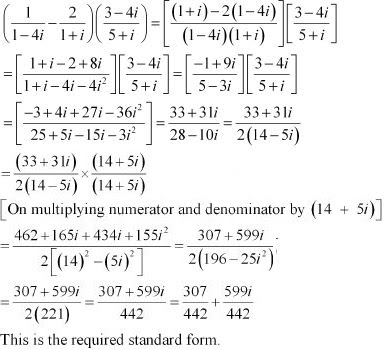4. If x - iy = √(a - ib)/(c-id) prove that (x2 + y2)2  = (a2 + b2)/(c2 + d2
Solution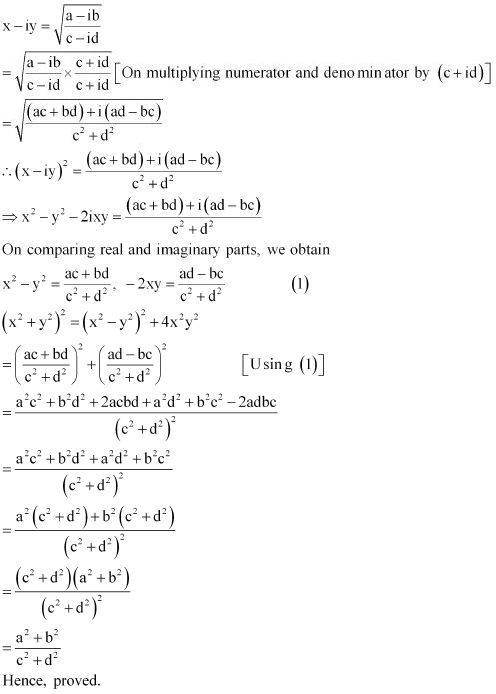5. Convert the following in the polar form :
(i) (1 + 7i)/(2 - i)2 ,
(ii) (1 + 3i)/(1 - 2i)
Solution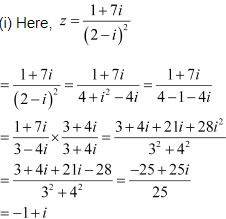Let r cos θ = –1 and r sin θ = 1
On squaring and adding, we obtain
r2 (cos2 θ + sin2 θ) = 1 + 1
⇒ r2 (cos2 θ + sin2 θ) = 2
⇒ r2 = 2  [cos2 θ + sin2 θ = 1]
⇒ r = √2  [Conventionally, r > 0]
∴ √2 cosθ = -1 and √2 sinθ = 1
⇒ cos θ = -1/√2 and sin θ = 1/√2
∴ θ = π - π/4 = 3π/4   [As θ lies in II quadrant]
∴ z = r cos θ + i r sin θ
= √2 cos (3π/4) + i √2sin (3π/4) = √2[ cos(3π/4) + i sin(3π/4)
This is the required polar form.

(ii) Here, z = (1 + 3i)/(1 - 2i)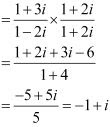Let r cos θ = –1 and r sin θ = 1
On squaring and adding, we obtain
r2 (cos2 θ + sin2 θ) = 1 + 1
⇒r2 (cos2 θ + sin2 θ) = 2
⇒ r2 = 2 [cos2 θ + sin2 θ = 1]
⇒ r = √2   [Conventionally, r > 0]
∴ √2 cosθ = -1 and √2sinθ = 1
⇒ cos θ = -1/√2 and sinθ = 1/√2
∴ θ = π - π4 = 3π/4  [As θ lies in II quadrant]
∴ z = r cos θ + i r sin θ
= √2 cos 3π/4 + i√2 sin3π/4 = √2 [cos (3π/4 + i sin(3π/4)]
This is the required polar form.

6. Solve the equation 3x2 - 4x + 20/3 = 0
Solution
The given quadratic equation is 3x2 - 4x + 20/3 = 0
This equation can also be written as 9x2 - 12x + 20 = 0
On comparing this equation with ax2 + bx + c = 0 , we obtain
a = 9 b = -12 and c = 20
Therefore, the discriminant of the given equation is
D = b2 - 4ac = (-12)2 - 4× 9 × 20 = 144 - 720 = -576
Therefore, the required solutions are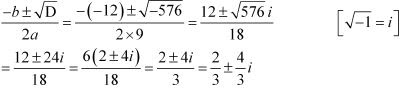7. Solve the equation x2 - 2x + 3/2 = 0
Solution
The given quadratic equation is x2 - 2x + 3/2 = 0
This equation can also be written as 2x2 - 4x +3 = 0
On comparing this equation with ax2 + bx + c = 0 we obtain
a = 2, b = -4 and c = 3
Therefore, the discriminant of the given equation is
D = b2 - 4ac = (-4)2 - 4 × 2 × 3 = 16 - 24 = -8
Therefore, the required solutions are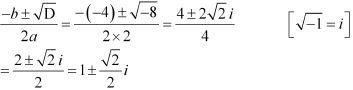8. Solve the equation 27x2 - 10x + 1 = 0
The given quadratic equation is 27x2 - 10x + 1 = 0
On comparing the given equation with ax2 + bx + c = 0, we obtain
a = 27, b = -10, and c = 1
Therefore, the discriminant of the given equation is
D = b2 - 4ac = (-10)2 - 4 × 27 × 1 = 100 - 108 = -8
Therefore, the required solutions are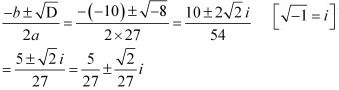9. Solve the equation 21x2 - 28x + 10 = 0
Solution
The given quadratic equation is 21x2 - 28x + 10 = 0
On comparing the given equation with ax2 + bx + c = 0 , we obtain
a = 21, b = -28 and c = 10
therefore, the discriminant of the given equation is
D = b2 - 4ac = (-28)2 - 4×21 ×10 = 784 - 840 = -56
Therefore, the required solutions are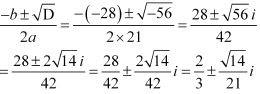10. If z1 = 2 – i , z2 = 1+ i , find |(z1 + z2 + 1)/(z1 – z­2 + 1)|
Solution
z1 = 2 – I , z2 = 1+ i ,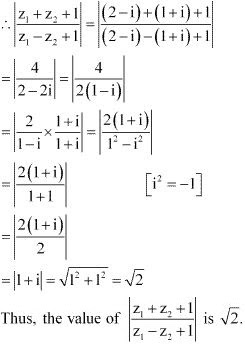11. If (a + ib) = (x + 1)2 /(2x2 + 1) , prove that a2 + b2 = (x2 + 1)2 /(2x + 1)2
Solution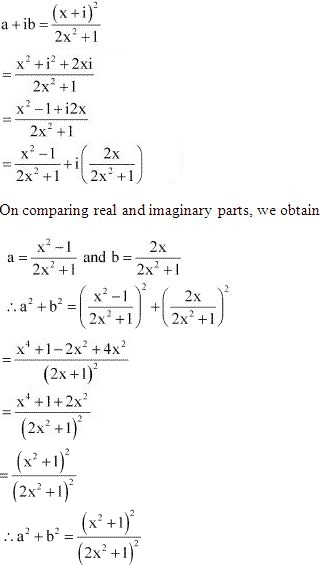12. Let z1 = 2 – i , z2 = -2+ i . find
(i) Re(z1 z2 /z1
(ii) Im(1/z1 z1)
Solution
z1 = 2 – i , z2 = -2+ i

(i) z1 z2 = (2 - i)(-2 + i) = -4 + 2i +2i - i2  = -4 + 4i -(-1) = -3 + 4i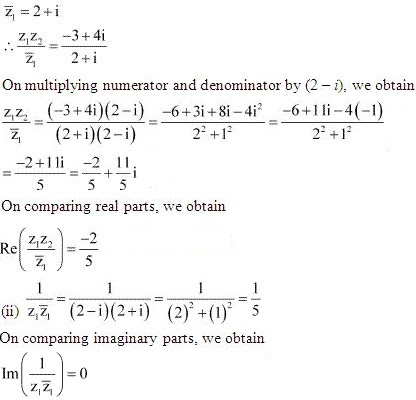13. Find the modulus and argument of the complex number (1 + 2i)/(1 - 3i).
Solution
Let z = (1 + 2i)/(1 - 3i) , then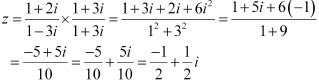Let z = r cos θ = -1/2 and r sin θ = 1/2
On squaring and adding, we obtain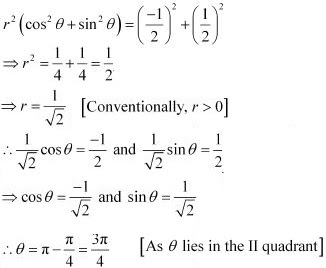14. Find the real numbers x and y if (x – iy) (3 + 5i) is the conjugate of –6 – 24i.
Solution
Let z = (x - iy)(3 + 5i)
z = 3x + 5xi - 3yi - 5yi2 = 3x + 5xi - 3yi + 5y = (3x + 5y) + i(5x - 3y)
∴ z  = (3x + 5y) - i(5x - 3y)
It is given that ,  z  = -6-24i
∴ (3x +5y) - i(5x - 3y) = -6 - 24i
Equating real and imaginary parts, we obtain
3x + 5y = -6 ...(i)
5x - 3y = 24 ...(ii)
Multiplying equation (i) by 3 and equation (ii) by 5 and then adding them, we obtain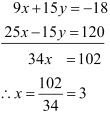Putting the value of x in equation (i), we obtain
3(3) + 5y = -6
⇒ 5y = -6 - 9 = -15
⇒ y = -3
Thus, the values of x and y are 3 and -3  respectively.

16. Find the modulus of [(1+i)/(1-i)] -[(1 -i)/(1 +i)].
Solution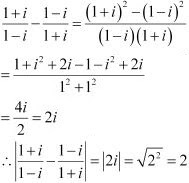17. If α and β are different complex numbers with |β| = 1, then find |(β - α)/(1 - α β)|.
Solution
Let α = a + ib and β = x +iy
It is given that, |β| = 1
∴ √(x2 + y2 ) = 1
⇒ x2  + y2  = 1 ...(i)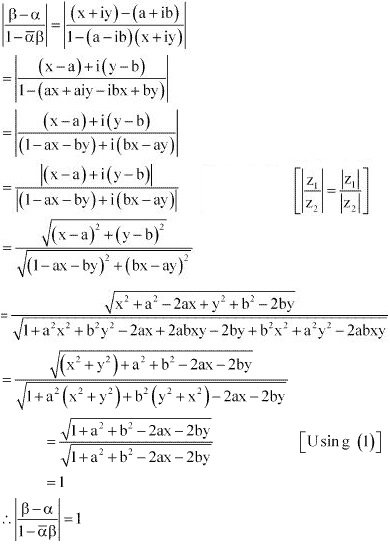18. Find the number of non-zero integral solutions of the equation |1-i|x  =  2x .
Solution
|1-x|x = 2x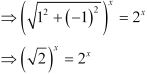⇒ 2x/2 = 2x
⇒ x/2 = x
⇒ x = 2x
⇒ 2x - x = 0
⇒ x = 0
Thus, 0 is the only integral solution of the given equation. Therefore, the number of non- zero integral solutions of the given equation is 0.

19. If (a + ib)(c + id)(e + if)(g + ih) = A + iB, then show that
(a2 + b2)(c2 + d2)(e2 + f2 )(g2 + h2) = A2 + B2
Solution
(a + ib)(c + id)(e + if)(g + ih) = A + iB
∴ |(a + ib)(c + id)(e + if)(g + ih)| = |A + iB|
⇒ |(a + ib)|× |(c + id)|× |(e + if)| × |(g + ih)| = |A + B|  [|z1 z2| z1||z2|]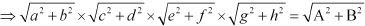On squaring both sides, we obtain
(a2 + b2 )(c2 + d2 )(e2 + f2 )(g2 + h2 ) = A2 + B2 .
Hence, proved.

20. If [(1 +i)/(1 - i)]m = 1, then find the least positive integral value of m.
Solution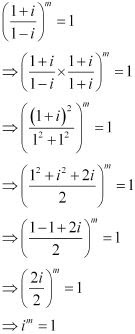∴ m = 4k, where k is some integer.
Therefore, the least positive integer is 1.
Thus, the least positive integral value of m is 4 (= 4× 1).